# Derivation in a ring

(diff) ← Older revision | Latest revision (diff) | Newer revision → (diff)

A mappingof a ringinto itself which is an endomorphism of the additive group ofand satisfies the relationLetbe a left-module. A derivation inwith values inis a homomorphism of the respective additive groups which satisfies the condition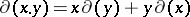for all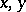from. For any elementfrom the centreof, the mapping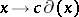, whereis a derivation, is a derivation. The sum of two derivations is also a derivation. This defines the structure of a-module on the set of all derivations inwith values in, denoted by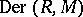. Ifis a subring in, a derivation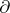such thatfor allis known as an-derivation. The set of all-derivations forms a submodule in, denoted by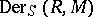. The operation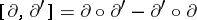defines the structure of a Lie-algebra on the-module. Ifis a homomorphism of-modules, then the composition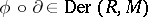for any.

Letbe a ring of polynomialswith coefficients in a commutative ring. The mapping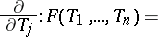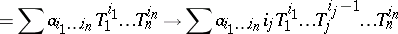is an-derivation in, and the-module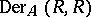is a free module with basis.

For any elementof an associative ring (or a Lie algebra)the mapping(or) is a derivation in, known as an inner derivation. Derivations which are not inner are known as outer.

Ifis a subring of a ring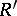and if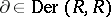, one says that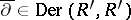is an extension ofif the restriction of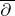tocoincides with. Ifis a commutative integral ring andis its field of fractions, and also ifis a separable algebraic extension of the fieldor ifis a Lie algebra over a fieldandis its enveloping algebra, there exists a unique extension of any derivation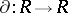to.

There is a close connection between derivations and ring isomorphisms. Thus, ifis a nilpotent derivation, that is, for some,, andis an algebra over a field of characteristic zero, the mapping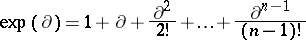is an automorphism of the-algebra. Ifis a local commutative ring with maximal ideal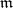, there is a bijection between the set of derivations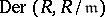and the set of automorphisms of the ring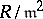which induces the identity automorphism of the residue field. Derivations of non-separable field extensions play the role of elements of the Galois group of separable extensions in the Galois theory of such extensions .

How to Cite This Entry:
Derivation in a ring. Encyclopedia of Mathematics. URL: http://encyclopediaofmath.org/index.php?title=Derivation_in_a_ring&oldid=17037
This article was adapted from an original article by I.V. Dolgachev (originator), which appeared in Encyclopedia of Mathematics - ISBN 1402006098. See original article